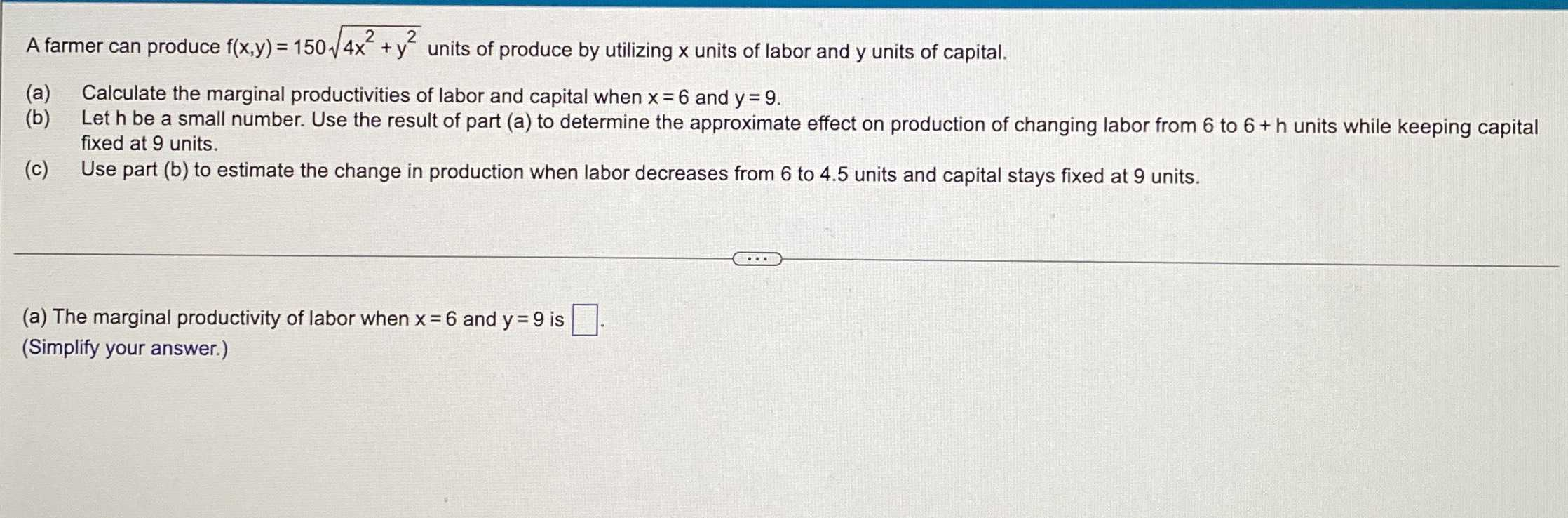### ¿Todavía tienes preguntas de matemáticas?

Pregunte a nuestros tutores expertos
Algebra
PreguntaA farmer can produce $$f ( x , y ) = 150 \sqrt { 4 x ^ { 2 } + y ^ { 2 } }$$ units of produce by utilizing $$x$$ units of labor and $$y$$ units of capital.

(a) Calculate the marginal productivities of labor and capital when $$x = 6$$ and $$y = 9$$ .

(b) Let $$h$$ be a small number. Use the result of part (a) to determine the approximate effect on production of changing labor from $$6$$ to $$6$$ + $$h$$ units while keeping capital fixed at $$9$$ units.

(c) Use part (b) to estimate the change in production when labor decreases from $$6$$ to $$4.5$$ units and capital stays fixed at $$9$$ units.

(a) The marginal productivity of labor when $$x = 6$$ and $$y = 9$$ is $$\square$$ . (Simplify your answer.)